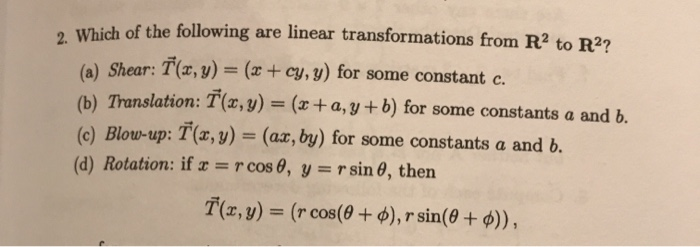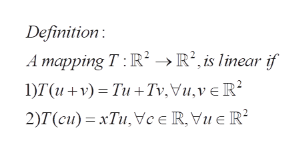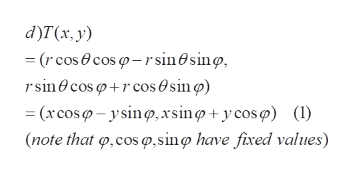# Which of the following are linear transformations from R2 to R2?(a) Shear: T(x, y) = (x+ cy, y) for some constant c.(b) Translation: T(x, y) = (x + a, y + b) for some constantsa and b.(c) Blow-up: T(r, y) = (ax, by) for some constants a and b.(d) Rotation: if r = r cos 0, y = rsin 0, thenT(z,y) = (r cos(60 + ), r sin(0 + )),

Question
14 views

Nedd help with d)help_outlineImage TranscriptioncloseWhich of the following are linear transformations from R2 to R2? (a) Shear: T(x, y) = (x+ cy, y) for some constant c. (b) Translation: T(x, y) = (x + a, y + b) for some constantsa and b. (c) Blow-up: T(r, y) = (ax, by) for some constants a and b. (d) Rotation: if r = r cos 0, y = rsin 0, then T(z,y) = (r cos(60 + ), r sin(0 + )), fullscreen
check_circle

Step 1

(d) To determine if the given mapping T is a linear transformation on the plane R^2

Step 2

Recall the definition of a linear transformation. We will show that a rotation as defined in d) is indeed a linear transfromation by verifying 1) and 2)help_outlineImage TranscriptioncloseDefinition: A mapping T: R->R2,is linear if 1)T(u+v) Tu+Tv.Vu,ve R 2)T(cu) xTu,ce R, Vu e R fullscreen
Step 3

d) write the mapping in terms of th...help_outlineImage Transcriptionclosed)T(x, y) (r cos cos -r sin0sinø rsin0cos rcos@sin p (rcos-ysin,xsinp+ycos ) () (note that o,cos p,sino have fixed values) fullscreen

### Want to see the full answer?

See Solution

#### Want to see this answer and more?

Solutions are written by subject experts who are available 24/7. Questions are typically answered within 1 hour.*

See Solution
*Response times may vary by subject and question.
Tagged in

### Math